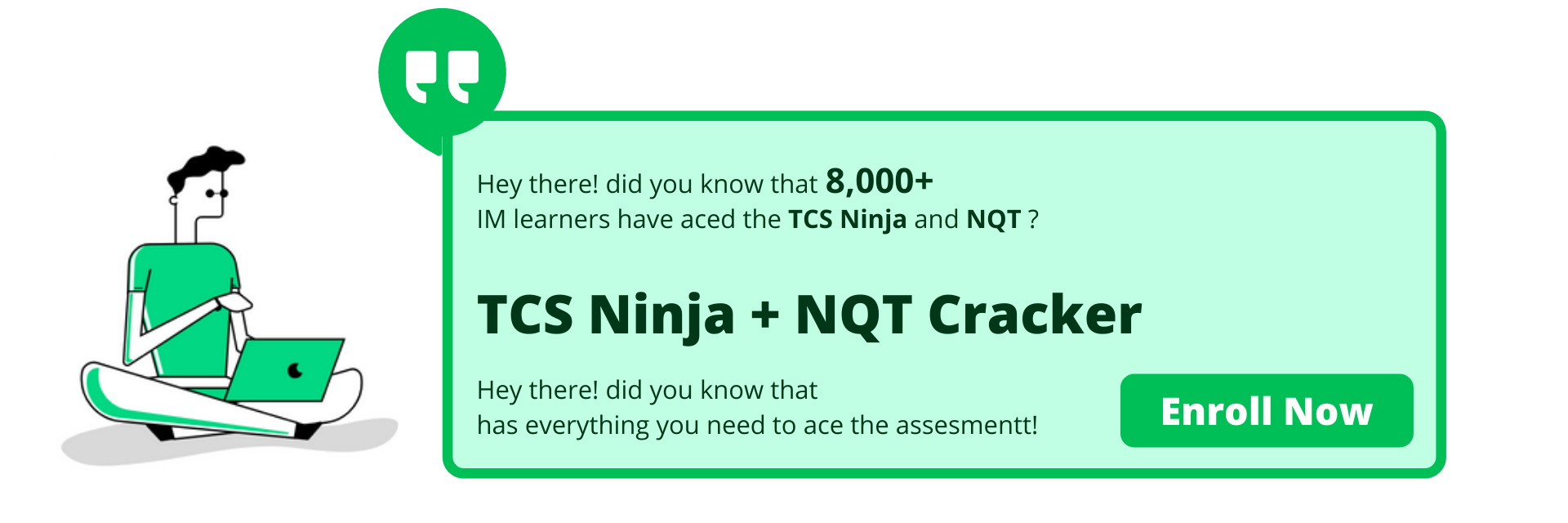# Top 150 Coding Problems## IlluminateMinds Top 150 Codes

Below You will find some of the most important codes in languages like C, C++ and Java. These codes are of prime importance for college semester exams and also for various online tests and interviews of the companies offering placements within varying range in LPA.

These codes are very important since these will help you clear your basic concepts in various languages.Prime-banner-1
Prime-banner-1
Prime-banner-1
Prime-banner-1## Coding Problem on Numbers

 Positive or Negative number: Even or Odd number: Power of a number : Factor of a number : Perfect number : Sum of First N Natural numbers: Sum of N natural numbers: Sum of numbers in a given range: Greatest of two numbers: Greatest of the Three numbers: Fibonacci Series upto nth term : Find the Nth Term of the Fibonacci Series : Prime number: Armstrong number : Armstrong number in a given range : Palindrome number: Prime number within a given range: Sum of digits of a number: Reverse of a number : Automorphic number : Factorial of a number : Finding Prime Factors of a number : Abundant number : Strong number : Harshad number : Friendly pair : Highest Common Factor(HCF): Lowest Common Multiple (LCM) : Hexadecimal to Decimal conversion : Decimal to Binary conversion : Greatest Common Divisor : Binary to Decimal to conversion : Octal to Decimal conversion : Maximum number of handshakes : Decimal to Octal Conversion : Octal to Binary conversion : Quadrants in which a given coordinate lies : Permutations in which n people can occupy r seats in a classroom : Replace all 0’s with 1 in a given integer : Addition of two fractions : Decimal to Hexadecimal Conversion : Binary to Octal conversion : Finding Number of times x digit occurs in a given input : Can a number be expressed as a sum of two prime numbers : Count possible decoding of a given digit sequence : Finding Roots of a quadratic equation : Check whether a character is a alphabet or not : Calculate the area of a circle : Find the ASCII value of a character : Check whether a character is a vowel or consonant : Calculate the number of digits in an integer : Convert digit/number to words : Counting number of days in a given month of a year : Find the prime numbers between 1 to 100 : Finding number of integers which has exactly x divisors :